Department of Pre-University Education, KarnatakaPUC Karnataka Science Class 12

# Question Bank Solutions for PUC Karnataka Science Class 12 - Department of Pre-University Education, Karnataka - Physics

Subjects
Topics
Subjects
Popular subjects
Topics
Physics
< prev  1 to 20 of 1916  next >

A circular coil of cross-sectional area 200 cm2 and 20 turns is rotated about the vertical diameter with angular speed of 50 rad s−1 in a uniform magnetic field of magnitude 3.0 × 10−2T. Calculate the maximum value of the current in the coil.

[0.07] Electromagnetic Induction
Chapter: [0.07] Electromagnetic Induction
Concept: Electromagnetic Induction

Show diagrammatically how an alternating emf is generated by a loop of wire rotating in a magnetic field. Write the expression for the instantaneous value of the emf induced in the rotating loop.

[0.07] Electromagnetic Induction
Chapter: [0.07] Electromagnetic Induction
Concept: Electromagnetic Induction

The coil of a moving-coil galvanometer keeps on oscillating for a long time if it is deflected and released. If the ends of the coil are connected together, the oscillation stops at once. Explain.

[0.07] Electromagnetic Induction
Chapter: [0.07] Electromagnetic Induction
Concept: Electromagnetic Induction

Consider the energy density in a solenoid at its centre and that near its ends. Which of the two is greater?

[0.07] Electromagnetic Induction
Chapter: [0.07] Electromagnetic Induction
Concept: Electromagnetic Induction

A conducting square loop of side l and resistance R moves in its plane with a uniform velocity v perpendicular to one of its sides. A uniform and constant magnetic field Bexists along the perpendicular to the plane of the loop as shown in figure. The current induced in the loop is _____________ .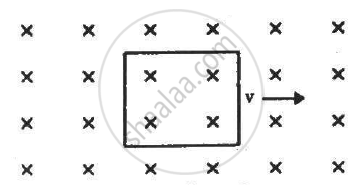[0.07] Electromagnetic Induction
Chapter: [0.07] Electromagnetic Induction
Concept: Electromagnetic Induction

A conducting rod is moved with a constant velocity v in a magnetic field. A potential difference appears across the two ends _____________ .

[0.07] Electromagnetic Induction
Chapter: [0.07] Electromagnetic Induction
Concept: Electromagnetic Induction

L, C and R represent the physical quantities inductance, capacitance and resistance respectively. Which of the following combinations have dimensions of frequency?

(a) 1/(RC)

(b) R/L

(c) 1/sqrt(LC)

(d) C/L

[0.07] Electromagnetic Induction
Chapter: [0.07] Electromagnetic Induction
Concept: Electromagnetic Induction

The switches in figure (a) and (b) are closed at t = 0 and reopened after a long time at t = t0.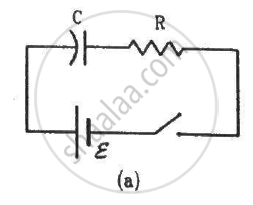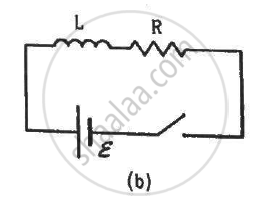(a) The charge on C just after t = 0 is εC.
(b) The charge on C long after t = 0 is εC.
(c) The current in L just before t = t0 is ε/R.
(d) The current in L long after t = t0 is ε/R.

[0.07] Electromagnetic Induction
Chapter: [0.07] Electromagnetic Induction
Concept: Electromagnetic Induction

Calculate the dimensions of (a) $\int \overrightarrow{E} . d \overrightarrow{l,}$ (b) vBl and (c) $\frac{d \Phi_B}{dt}.$ The symbols have their usual meaning.

[0.07] Electromagnetic Induction
Chapter: [0.07] Electromagnetic Induction
Concept: Electromagnetic Induction

A conducting square loop having edges of length 2.0 cm is rotated through 180° about a diagonal in 0.20 s. A magnetic field B exists in the region which is perpendicular to the loop in its initial position. If the average induced emf during the rotation is 20 mV, find the magnitude of the magnetic field.

[0.07] Electromagnetic Induction
Chapter: [0.07] Electromagnetic Induction
Concept: Electromagnetic Induction

Figure shows a wire sliding on two parallel, conducting rails placed at a separation l. A magnetic field B exists in a direction perpendicular to the plane of the rails. What force is necessary to keep the wire moving at a constant velocity v ?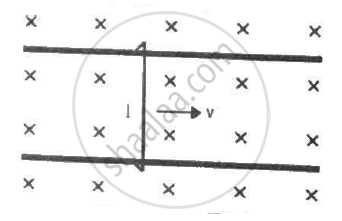[0.07] Electromagnetic Induction
Chapter: [0.07] Electromagnetic Induction
Concept: Electromagnetic Induction

Figure shows a long U-shaped wire of width l placed in a perpendicular magnetic field B. A wire of length l is slid on the U-shaped wire with a constant velocity v towards right. The resistance of all the wires is r per unit length. At t = 0, the sliding wire is close to the left edge of the U-shaped wire. (a) Calculate the force needed to keep the sliding wire moving with a constant velocity v. (b) If the force needed just after t = 0 is F0, find the time at which the force needed will be F0/2.0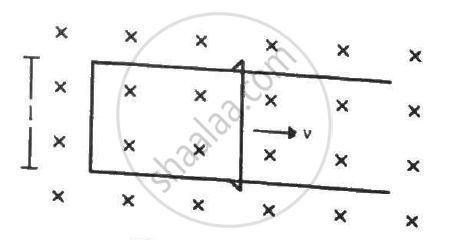[0.07] Electromagnetic Induction
Chapter: [0.07] Electromagnetic Induction
Concept: Electromagnetic Induction
Why do you mean by turns ratio of a transformer? Can it work with D.C.?
[0.07] Electromagnetic Induction
Chapter: [0.07] Electromagnetic Induction
Concept: Electromagnetic Induction

Why does a compass needle get deflected when brought near a bar magnet?

[0.04] Magnetism and Matter
Chapter: [0.04] Magnetism and Matter
Concept: Introduction of Magnetism

Draw a labelled diagram of an ac generator.

[0.07] Electromagnetic Induction
Chapter: [0.07] Electromagnetic Induction
Concept: AC Generator

Obtain the expression for the emf induced in the rotating coil of N turns each of cross-sectional area A, in the presence of a magnetic field vecB .

[0.07] Electromagnetic Induction
Chapter: [0.07] Electromagnetic Induction
Concept: AC Generator

Why should the objective of a telescope have large focal length and large aperture? Justify your answer.

[0.09] Ray Optics and Optical Instruments
Chapter: [0.09] Ray Optics and Optical Instruments
Concept: Optical Instruments > Telescope

Draw a ray diagram depicting the formation of the image by an astronomical telescope in normal adjustment.

[0.09] Ray Optics and Optical Instruments
Chapter: [0.09] Ray Optics and Optical Instruments
Concept: Optical Instruments > Telescope

You are given the following three lenses. Which two lenses will you use as an eyepiece and as an objective to construct an astronomical telescope ? Give reason

 Lenses Power (D) Aperture (cm) L1 3 8 L2 6 1 L3 10 1
[0.09] Ray Optics and Optical Instruments
Chapter: [0.09] Ray Optics and Optical Instruments
Concept: Optical Instruments > Telescope

In the figure given below the input waveform is converted into the output waveform by a device ‘X’. Name the device and draw its circuit diagram.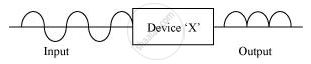[0.14] Semiconductor Electronics - Materials, Devices and Simple Circuits
Chapter: [0.14] Semiconductor Electronics - Materials, Devices and Simple Circuits
Concept: Integrated Circuits
< prev  1 to 20 of 1916  next >